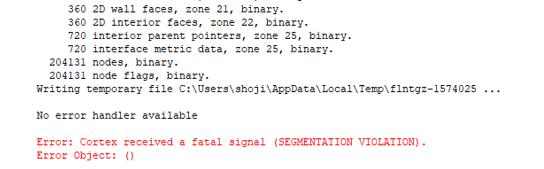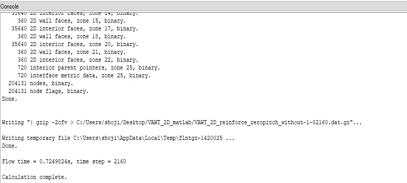## Fluids

•shoji
Subscriber

I've got the fluent error and I can't find why it occurs. If you have the solution, please tell me. Any advise would be appreciate.

I'm trying to optimize the wind turbine by combining ANSYS fluent and MATLAB(for genetic algorithm) . Before optimizing wind turbine, I checked if Fluent operate normally by using TUI(text user interface) from MATLAB. To do it, I made simple MATLAB code as follows. Four lines from the top is code for connecting MATLAB and fluent, and fifth line make fluent read case file, sixth line make fluent start calculation.

orb=initialize_orb()

iCoFluentUnit=actfluentserver(orb,'aaS_FluentId.txt');

iFluentTuiInterpreter=iCoFluentUnit.getSchemeControllerInstance();

When I execute this command from MATLAB, fluent starts calculation normally and torque data was output after the calculation terminated. However, the error message was displayed on the fluent console at  end of the calculation like this.When I start same calculation from fluent (not using MATLAB), error didn't occur as follows.Besides, when I tried calculating fewer time steps such as 400 ( ordered from MATLAB), error didn't occur.

In short,the error only occurs when ordered from MATLAB and time step is bigger than specific value. The error doesn't happen when I calculate without using MATLAB or fewer time steps than specific value.

•shoji
Subscriber

?OS : Windows10(64bit)

?Graphic card : Intel UHD Graphics 630

?Processor : Intel Corei7-8700 3.20Ghz

?Memory : 16384MB RAM

?CFD soft : ANSYS Fluent 17.2

?MATLAB R2018b